Courses

# Metallurgical Engineering - MT 2015 GATE Paper (Practice Test)

## 65 Questions MCQ Test GATE Past Year Papers for Practice (All Branches) | Metallurgical Engineering - MT 2015 GATE Paper (Practice Test)

Description
This mock test of Metallurgical Engineering - MT 2015 GATE Paper (Practice Test) for GATE helps you for every GATE entrance exam. This contains 65 Multiple Choice Questions for GATE Metallurgical Engineering - MT 2015 GATE Paper (Practice Test) (mcq) to study with solutions a complete question bank. The solved questions answers in this Metallurgical Engineering - MT 2015 GATE Paper (Practice Test) quiz give you a good mix of easy questions and tough questions. GATE students definitely take this Metallurgical Engineering - MT 2015 GATE Paper (Practice Test) exercise for a better result in the exam. You can find other Metallurgical Engineering - MT 2015 GATE Paper (Practice Test) extra questions, long questions & short questions for GATE on EduRev as well by searching above.
QUESTION: 1

Solution:
QUESTION: 2

Solution:
QUESTION: 3

### Choose the statement where underlined word is used correctly.

Solution:
QUESTION: 4

Tanya  is older than Eric.

Cliff is older than Tanya.

Eric is older than Cliff.

if the first two statements are true, then the third statement is :

Solution:
QUESTION: 5

Five teams have to complete in a league, with every team playing every other team exactly once, before going to the next round. How many matches will have to be held to complete the league round of matches?

Solution:
QUESTION: 6

Q.6-10 Carry two Mark each

Select the appropriate option in place of underlined part of the sentence.

"Increased productivity necessary" reflects greater efforts made by the employees.

Solution:
QUESTION: 7

Given below are two statements followed by two conclusions. Assuming these statements to be true, decide  which one logically follows.

Statements:

I. No manager is a leader.

Conclusions:

II. No executive is a manager.

Solution:
*Answer can only contain numeric values
QUESTION: 8

In the given figure angle Q is a right angles, PS:QS= 3:1, RT:QT = 5:2 and PU:UR = 1:1. If area of triangle QTS is 20 cm2, then the area of triangle PQR in cmis____________.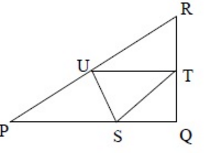Solution:
QUESTION: 9

Right triangle PQR is to be constructed in the xy- plane so that the right angle is at P and line PR is parallel to the x- axis. The x and y coordinates of P,Q and R are to be integers that satisfy the inequalities; -4< x< 5 and 6< y< 16. How many different triangles could be constructed with these properties?

Solution:
QUESTION: 10

A coin is tossed thrice. Let X be the event that head occurs in each of the first two tosses. Let Y be the event that a tail occurs on the thrid toss. Let Z be the event that two tails occur in the three tosses. Based on the above information, which one of the following statements is True?

Solution:
*Answer can only contain numeric values
QUESTION: 11

Q. 11 – Q. 35 carry ONE mark each

Consider the following five readings from an experiment: 19, 17, 15, 13, 11. The standard deviation of the readings is________.

Solution:
QUESTION: 12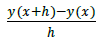is a numerical approximation for

Solution:
QUESTION: 13

If A and B are matrices, (A)T =

Solution:
QUESTION: 14

Which of the following properties is intensive?

Solution:
QUESTION: 15

In an Ellingham diagram, the standard free energy change ΔG° for the oxidation reaction of a metal M given by: xM(s) + O2(g)→ MxO2(s) , is plotted as a function of temperature. The slope of this line is positive because

Solution:
QUESTION: 16

In froth flotation, hydrophobic mineral particles ascend with air bubbles preferentially over hydrophilic mineral particles. The figure below shows a schematic of a water droplet placed on the surfaces of two mineral P and Q.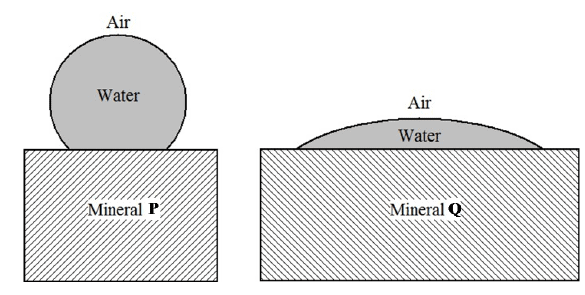Given this information, pick the CORRECT statement from the following:

Solution:
QUESTION: 17

Which of the following oxide addition results in polymerization (i.e., network formation) in a silicate slag?

Solution:
QUESTION: 18

Zn is commercially extracted from which of the following minerals?

Solution:
QUESTION: 19

Self supporting arches for furnace roofs can be fabricated using silica bricks but not using magnesia bricks. Why?

Solution:
QUESTION: 20

A species can diffuse through the lattice (diffusion coefficient, DL ), along grain boundaries (diffusion coefficient, DGB ), and along free surfaces (diffusion coefficient, DS ). Which of the following relations is CORRECT?

Solution:
QUESTION: 21

Select the CORRECT plot of Gibbs free energy (G) vs. temperature (T) for a single component system from the following: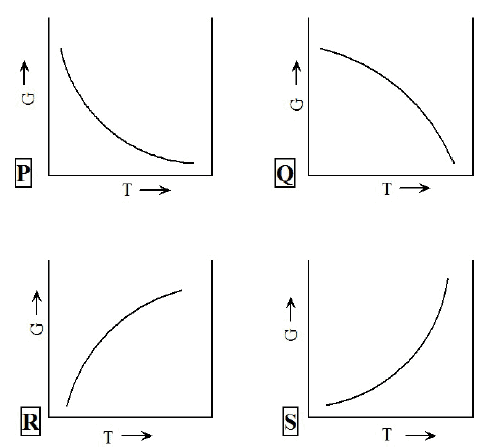Solution:
QUESTION: 22

If Δx represents adherent oxide layer thickness and t is time, which of the following curves represents diffusion-controlled oxidation kinetics?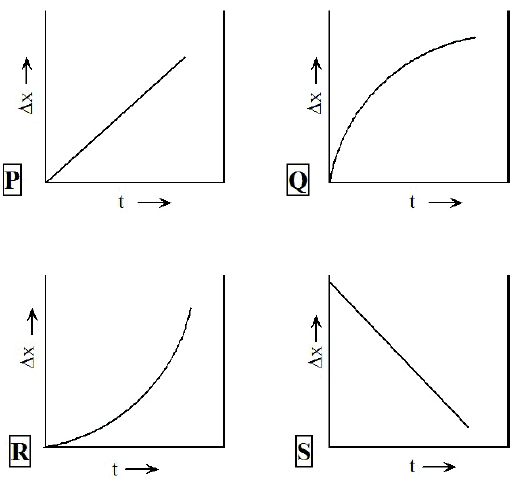Solution:
QUESTION: 23

Based on the standard galvanic series, select the CORRECT sequence of metals in the increasing order of anodic behaviour:

Solution:
QUESTION: 24

In a conventional unit cell of a crystal, a = b ≠ c and α= β = γ=90°. This crystal belongs to which of the following systems?

Solution:
QUESTION: 25

In an X-Ray powder pattern of a simple cubic crystal, the 2nd peak corresponds to

Solution:
QUESTION: 26

When boron (trivalent) is doped to silicon, the resulting material is

Solution:
QUESTION: 27

Which of the following metal working operations can be categorized as an indirect compression process?

Solution:
QUESTION: 28

Which of the following is a typical rolling defect?

Solution:
QUESTION: 29

Which of the following manufacturing processes is NOT used for producing fine grained metals?

Solution:
QUESTION: 30

Which of the following metal forming techniques is used to produce soft drink cans from aluminium sheets?

Solution:
QUESTION: 31

Which of the following is NOT a solid state metal joining technique?

Solution:
*Answer can only contain numeric values
QUESTION: 32

The stress required for Orowan dislocation bypass is 200 MPa in an alloy when the inter-precipitate spacing is 500 nm. In the same alloy, if the inter-precipitate spacing is reduced to 200 nm, the stress required (in MPa) is _____ .

Solution:
QUESTION: 33

Which of the following Mohr's circles of a plane-stress condition corresponds to equi-biaxial tension?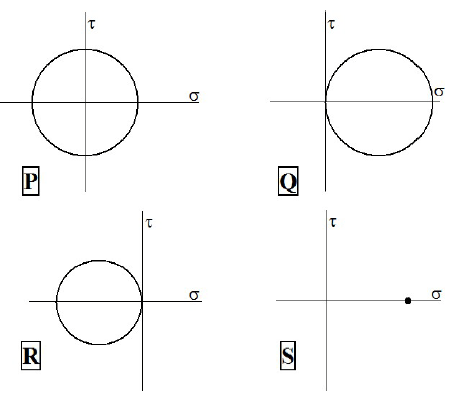Solution:
QUESTION: 34

Select the INCORRECT statement related to the effect of a small amount of carbon addition on mechanical properties of iron.

Solution:
QUESTION: 35

In polymers such as epoxies, creep resistance can be enhanced by

Solution:
*Answer can only contain numeric values
QUESTION: 36

Q. 36 – Q. 65 carry TWO marks each

One of the eigenvalues of the matrix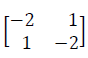is −3. The other eigenvalue is ______.

Solution:
*Answer can only contain numeric values
QUESTION: 37

Consider the scalar function f=xyz. The magnitude of the gradient, i.e.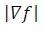at the point (0,2,2) is _________.

Solution:
*Answer can only contain numeric values
QUESTION: 38

The determinant of the matrix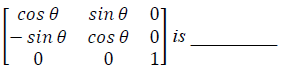Solution:
QUESTION: 39

The solution of the ordinary differential equation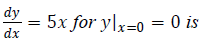Solution:
*Answer can only contain numeric values
QUESTION: 40

The maximum value of the function f(x)= −x2+2x is _________.

Solution:
QUESTION: 41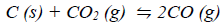is an important reaction in iron making.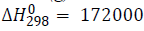joules per mole of CO2, which of the following conditions will favour the forward reaction?

Solution:
*Answer can only contain numeric values
QUESTION: 42

Consider the reaction: Fe3O4 (solid, pure) + CO (gas, 1 atm) →3FeO (solid, pure) + CO2 (gas, 1 atm)
For this reaction, ΔG°12000=−8000 joules per mole of CO and R = 8.314 J mol-1K-1.
The equilibrium ratio, pco2/pco for the reaction at 1200 K and 1 atm is ______

Solution:
*Answer can only contain numeric values
QUESTION: 43

An iron blast furnace produces hot metal containing 95% Fe. The iron ore charged into the furnace contains 95% Fe2O3 and the rest is gangue. Assume that all the iron in the ore goes to hot metal. The amount of iron ore (in kg) required for producing 1000 kg of hot metal is _______ .

(Atomic weight of Fe = 56 g mol-1 and that of Fe2O3 = 160 g mol-1)

Solution:
QUESTION: 44

The figure below shows water flowing through a pipe. The pressure difference between points P and Q measured using a water-over-mercury manometer is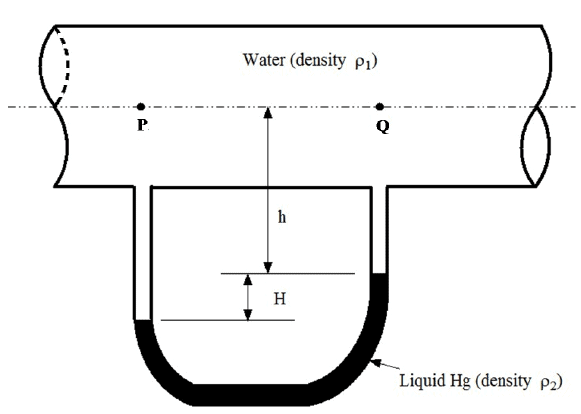Solution:
QUESTION: 45

Match the metals listed in Group I with the most appropriate extraction routes listed in Group II.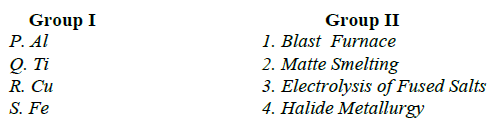Solution:
*Answer can only contain numeric values
QUESTION: 46

The figure below shows the cumulative size distribution of particles of a crushed mineral. 10 kg of this material is first passed through a sieve of size 400 micron and then through a sieve of size 300 micron. The weight of mineral (in kg) that is retained on the 300 micron sieve is ______ .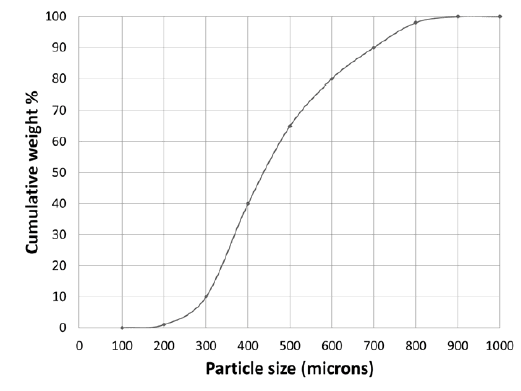Solution:
*Answer can only contain numeric values
QUESTION: 47

In electrolytic refining of Ni, the anode is Cu-10 atom % Ni and the cathode is pure Ni. Assuming the Cu-Ni solution to be ideal, the ABSOLUTE value of the minimum voltage (in mV) required for refining is ______ .

Given: Faraday constant = 96490 C mol-1, Temperature = 300 K, R = 8.314 J mol-1 K-1.

Solution:
*Answer can only contain numeric values
QUESTION: 48

Configurational entropy due to ideal mixing in a binary A-B system is expressed as:
ΔSmix =−R(XAln XA+XBln XB ), where XA and XB are mole fractions of A and B respectively. ΔSmix is maximum at XA =______

Solution:
*Answer can only contain numeric values
QUESTION: 49

Melting point of a metal is 1356 K. When the liquid metal is undercooled to 1256 K, the free energy change for solidification, ΔGL→S = −1000 J mol-1. On the other hand, if the liquid metal is undercooled to 1200 K, the free energy change (in J mol-1) for solidification is _____.

Solution:
QUESTION: 50

Match the names listed in Group I with the reactions listed in Group II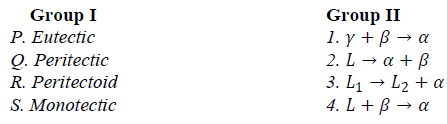Solution:
*Answer can only contain numeric values
QUESTION: 51

It takes 10 hours to homogenize an alloy at 1273 K. The time required (in hours) to achieve the same extent of homogenization at 1373 K is _____ .
Given: Diffusivity, D1373 K = 10−18m2 s-1 and D1273 K = 10−19m2 s-1

Solution:
QUESTION: 52

Match the materials listed in Group I with the most appropriate applications listed in Group II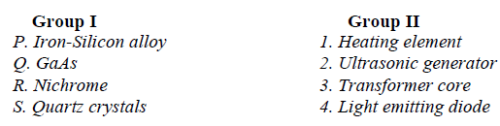Solution:
QUESTION: 53

Match the heat treatments for an eutectoid steel shown in the TTT diagram below (as P, Q, R and S) with the resulting microstructures listed below:

1. Fine pearlite

2. Martensite

3. Bainite

4. Coarse pearlite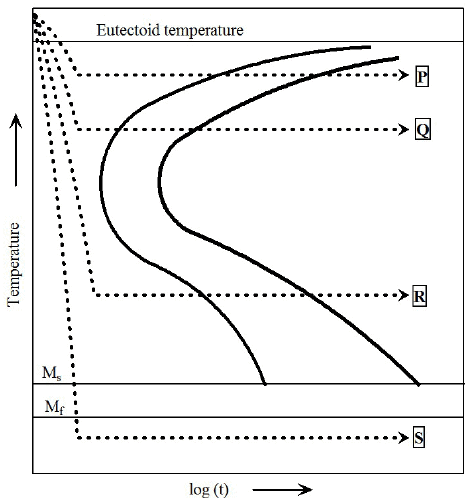Solution:
*Answer can only contain numeric values
QUESTION: 54

An alloy of overall composition XB=0.7 was equilibrated at temperature T1. Microstructural analysis showed two phases, α and β, and that the phase fraction of β was 0.75. Given that the equilibrium composition of β at T1 is 0.9 as shown in the phase diagram below, the maximum solubility of B in α (in mole fraction) at this temperature is _______.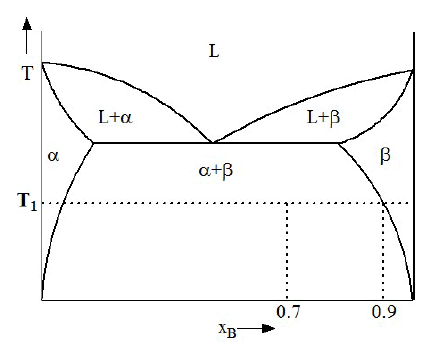Solution:
*Answer can only contain numeric values
QUESTION: 55

If a cylindrical billet of height 1.0 m and diameter 0.5 m is upset forged to form a cylindrical pancake of height 0.25 m, the diameter of the pancake (in m) is ______ .

Solution:
QUESTION: 56

Determine the correctness or otherwise of the following Assertion [a] and the Reason [r]

Assertion: In a pure metal weld, elastic modulus in the heat affected zone (HAZ) is the same as that in the base metal.

Reason: Coarse grained microstructure in the HAZ results in lower hardness.

Solution:
*Answer can only contain numeric values
QUESTION: 57

At the mould exit of a continuous caster, the metal consisting of a solidified shell with a liquid metal core exits at the rate of 35 kg s-1. Given that the latent heat of fusion is 3 × 105 J kg-1 and the total rate of heat removal by the mould is 4.2 × 106 W, the mass fraction of solid at the mould exit is _____.

Assume that both solid and liquid remain at the melting point while they are in the mould.

Solution:
QUESTION: 58

Match the features observed in castings listed in Group I with the most appropriate reasons listed in Group II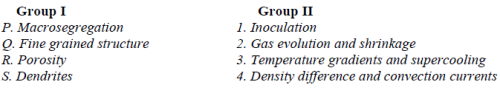Solution:
*Answer can only contain numeric values
QUESTION: 59

The driving force for sintering a compact consisting of spherical particles of radius R1 is ΔG1. If the particle size is reduced to R2 = 0.1 R1, the corresponding driving force ΔG2 = α ΔG1 , where α is _____ .

Solution:
QUESTION: 60

Which of the following techniques are NOT applicable for detecting internal flaws in a ceramic material?

1. Liquid penetration test

3. Ultrasonic testing

4. Eddy current method

Solution:
QUESTION: 61

Match the following fracture surface features listed in Group I with the fracture mechanisms listed in Group II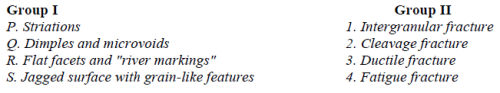Solution:
QUESTION: 62

Match the scientist pairs listed in Group I with phenomena listed in Group II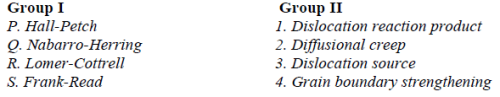Solution:
*Answer can only contain numeric values
QUESTION: 63

In an FCC crystal, the strain energy per unit length of a dislocation with Burgers vector a/2<110> is _____ times that of a a/6<112> dislocation.

Solution:
QUESTION: 64

Match the desired mechanical properties listed in Group I with the microstructural features listed in Group II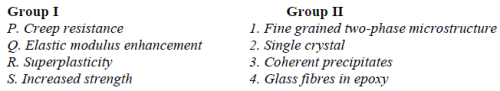Solution:
*Answer can only contain numeric values
QUESTION: 65

A brittle material is mechanically tested in medium P in which it has surface energy γs= 0.9 J m-2. This material has a fracture strength of 300 MPa for a given flaw size. The same solid containing the same flaws is then tested in medium Q in which γs= 0.1 J m-2. The fracture strength (in MPa) in medium Q based on Griffith's theory is _________ .

Solution: